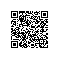# 变量名的命名规则

1. 仅仅由大、小写英文字母，下划线(_)，数字(不可作为变量名的开头)组合而成；
2. 不能使用Python关键字和函数名作为变量名；
3. 变量名不能包含空格，但可以使用_来分隔其中的单词；
4. 变量名应既简短又具有描述性；
5. 慎用字母lO，因为它们可能被人错看成数字1和0。

# python变量不需要声明变量类型

python规定，变量是没有类型之分的，变量的使用不需要提前声明和定义，只需对变量进行赋值，赋值的同时该变量即被创建。

Python是动态的，变量的类型由赋予它的值来决定。

a=2
type(a)
int
b=a
type(b)
int
a=2.5
type(a)
float
type(b)
int
print(a)
print(b)
2.5
2
b=b*a
print(b)
5.0

a='hello'
print(a)

a='world'
print(a)
hello
world

## 赋值语句(没有返回值)

### 增值变量

$$A+=B$$ 等价于 $$A=A+B$$
+可以换成-,*,/,%,**等符号。

### 变量的引用

1. 标识对象的类型的类型标识符；
2. 记录对象引用次数的计数器。

Python中变量是没有类型的，类型的概念是属于对象的。使用id()函数可以返回对象的内存地址。

n=7
id(n)
1973873296

# 赋值语句的使用原理：

1. 创建一个对象，前提是该对象未被创建。
2. 创建一个变量，前提是该变量未被创建。
3. 将对象的引用值赋给变量，引用器值增加1。

R=[2,4,5,8]
S=R
S
[2, 4, 5, 8]
R
[2, 4, 5, 8]
id(S)==id(R)
True
R=6
R
[2, 4, 6, 8]
S
[2, 4, 6, 8]
id(S)==id(R)
True

### 增量赋值与普通赋值的区别

• 二者对于不可变对象的处理方式见赋值语句的使用原理
• 对于可变对象，二者便会不同，增量赋值会将可变对象就地修改，而不是创建新的对象。

L=[1,2,3]
id(L)
2196737070792
L+=
id(L)
2196737070792
L=L+
id(L)
2196741665416

### 多重赋值

X=Y=Z=22
print(X)
print(Y)
print(Z)
22
22
22
X,Y,Z=2,"U",3.56
print(X)
print(Y)
print(Z)
2
U
3.56
Y,X=X,Y
print(X)
print(Y)
U
2使用钉钉扫一扫加入圈子
+ 订阅